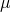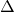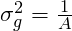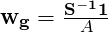## Calculating the Efficient Frontier: Part 2

Jun 142011
###### A Matrix Based Approach for Calculating Portfolio Weights

In a previous post, I showed how to calculate and plot the efficient frontier for a set of risky assets. In this post, I extend the previous example and show how to use Octave or Matlab to calculate the portfolio weights for each of the various risky assets for any point on the efficient frontier.

In my next post, I’ll conclude this series on the efficient frontier by adding a risk free asset,  showing the calculation for the tangency portfolio, and demonstrating how this creates a new frontier.

###### Calculating the Weights for Key Points on the Efficient Frontier

We can calculate the weights for any point on the efficient frontier once we know the weights for any two points on the efficient frontier.

Two easy points to calculate are the global minimum variance portfolio and the tangency portfolio for the case where the risk free rate is assumed to be zero.

Global Minimum Variance Portfolio

A point of particular interest on the efficient frontier is the “global minimum variance portfolio”.  This portfolio is the point on the efficient frontier which has the minimum variance or standard deviation.

We can solve for the mean and variance of the global minimum variance portfolio by setting the derivative of the equation for the variance to zero and solving for.  The equations for calculating the A,B,C andvalues are given in the previous post.Setting the derivative with respect toto zero gives:Running through all the algebra gives:Finally, the portfolio weights for the global minimum variance portfolio are given by: